﻿ 高速破片侵彻下防护液舱后板的载荷特性数值分析
 舰船科学技术2018, Vol. 40Issue (4): 1-5PDF

Numerical analysis on load characteristic of guarding liquid cabin′s back plate under high velocity fragment impact
WU Lin-jie, HOU Hai-liang, ZHU Xi
Department of Naval Architecture Engineering, Naval University of Engineering, Wuhan 430033, China
Abstract: Numerical simulations were conducted to research load characteristic of guarding liquid cabin′s back plate under high velocity fragment impact by using LS_DYNA software. The spatial distribution of liquid cabin′s back plate load was analyzed, as well as the effect on liquid cabin′s back plate load of fragment′s velocity and thickness. The fitting formulas for calculating peak pressure and specific impulse at any one point of liquid cabin′s back plate were gained. The research shows that, the load is ultimate at center point of liquid cabin′s back plate which is the projective point of fragment′s center point on the plate, and is exponentially declined when increasing the distance from the center point to the other one point. If fragment′s velocity or thickness increases, the peak pressure and specific impulse at any one point of liquid cabin′s back plate both increase.
Key words: high velocity fragment     guarding liquid cabin     load characteristic     numerical analysis
0 引　言

1 有限元数值计算 1.1 有限元模型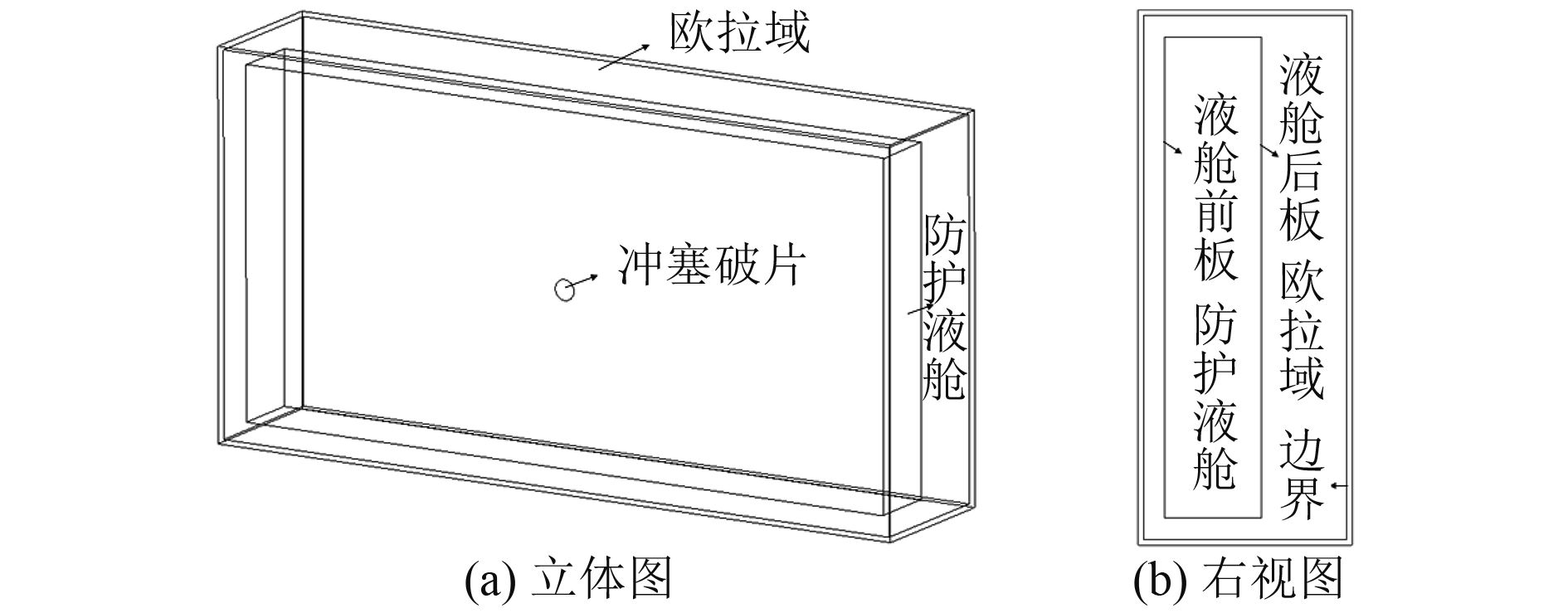图 1 有限元模型 Fig. 1 Finite element model
1.2 材料模型及参数

 $p = {C_0} + {C_1}\mu + {C_2}{\mu ^2} + {C_3}{\mu ^3} + ({C_4} + {C_5}\mu + {C_6}{\mu ^2})E\text{。}$ (1)

 $p \!=\! \frac{{{\rho _0}{C^2}\mu \left[ {1 \!+\! \left( {1 \!-\! \frac{{{\gamma _0}}}{2}} \right)\mu \!-\! \frac{\alpha }{2}{\mu ^2}} \right]}}{{{{\left[ {1 \!-\! \left( {{S_1} \!-\! 1} \right)\mu \!-\! {S_2}\frac{{{\mu ^2}}}{{\mu \!+\! 1}} \!-\! {S_3}\frac{{{\mu ^3}}}{{{{\left( {\mu \!+\! 1} \right)}^2}}}} \right]}^2}}} \!+\! \left( {{\gamma _0} \!+\! \alpha \mu } \right)E\text{。}$ (2)

 ${\sigma _d} = \left( {{\sigma _0} + \frac{{E{E_h}{\varepsilon _p}}}{{E - {E_h}}}} \right)\left[ {1 + {{\left( {\frac{{\dot \varepsilon }}{D}} \right)}^{\frac{1}{n}}}} \right]\text{。}$ (3)

1.3 压力测点及计算工况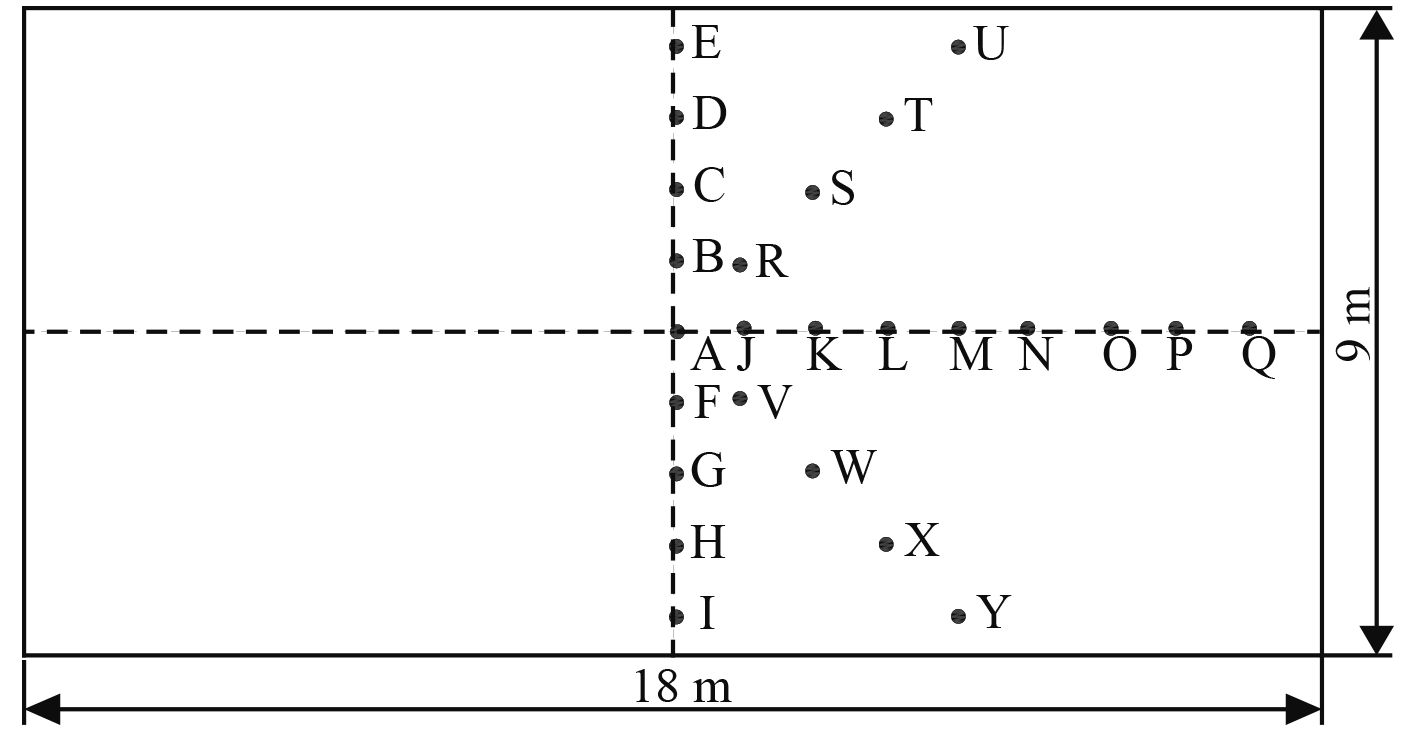图 2 液舱后板的压力测点 Fig. 2 The points on back plate of water cabin for outputting pressure表 1 计算工况 Tab.1 The calculation cases
2 计算结果及分析 2.1 工况1下防护液舱后板的载荷分析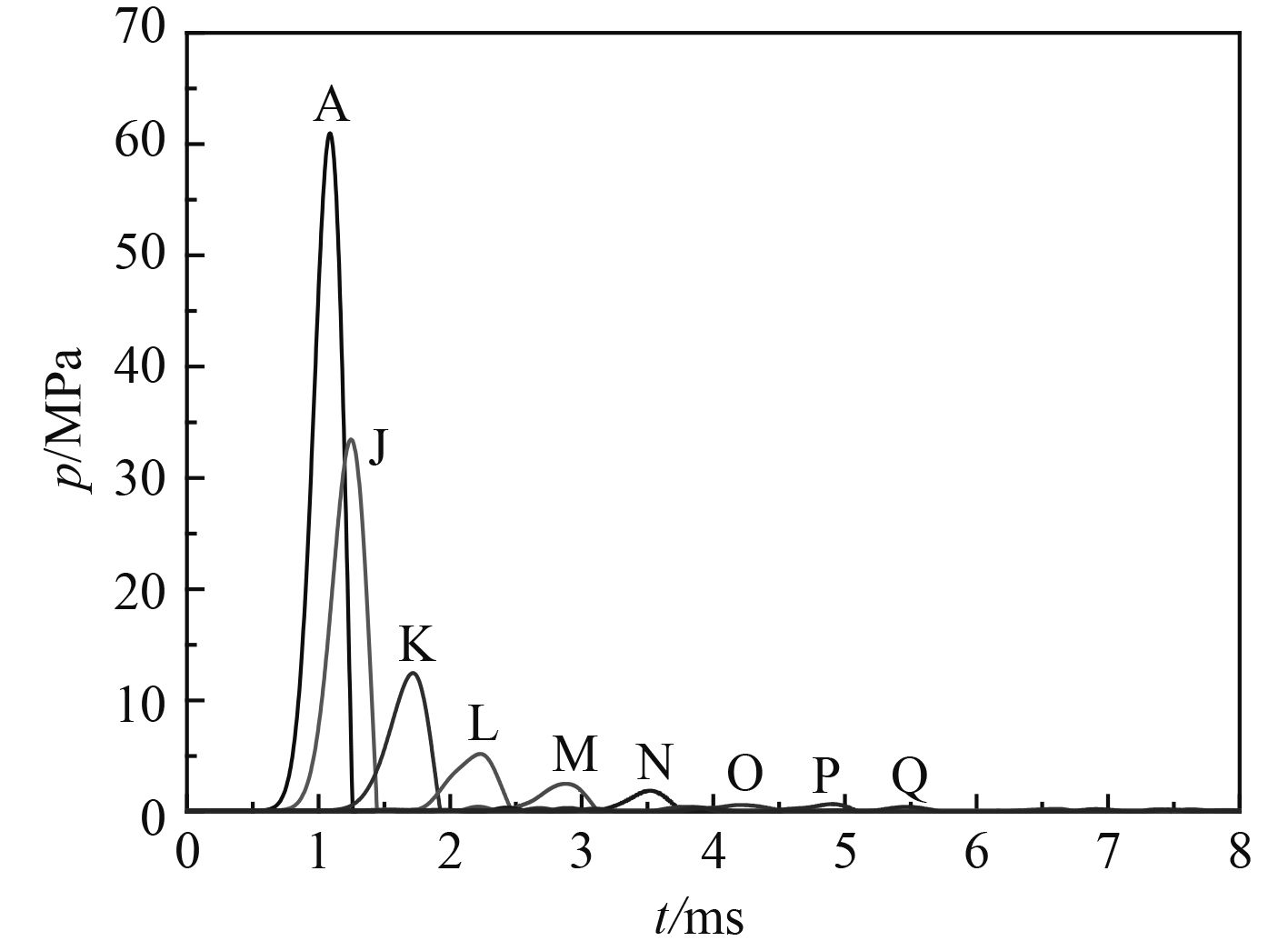图 3 工况1下测点A，J～Q的压力曲线 Fig. 3 The pressure curves of points A and J～Q in case 1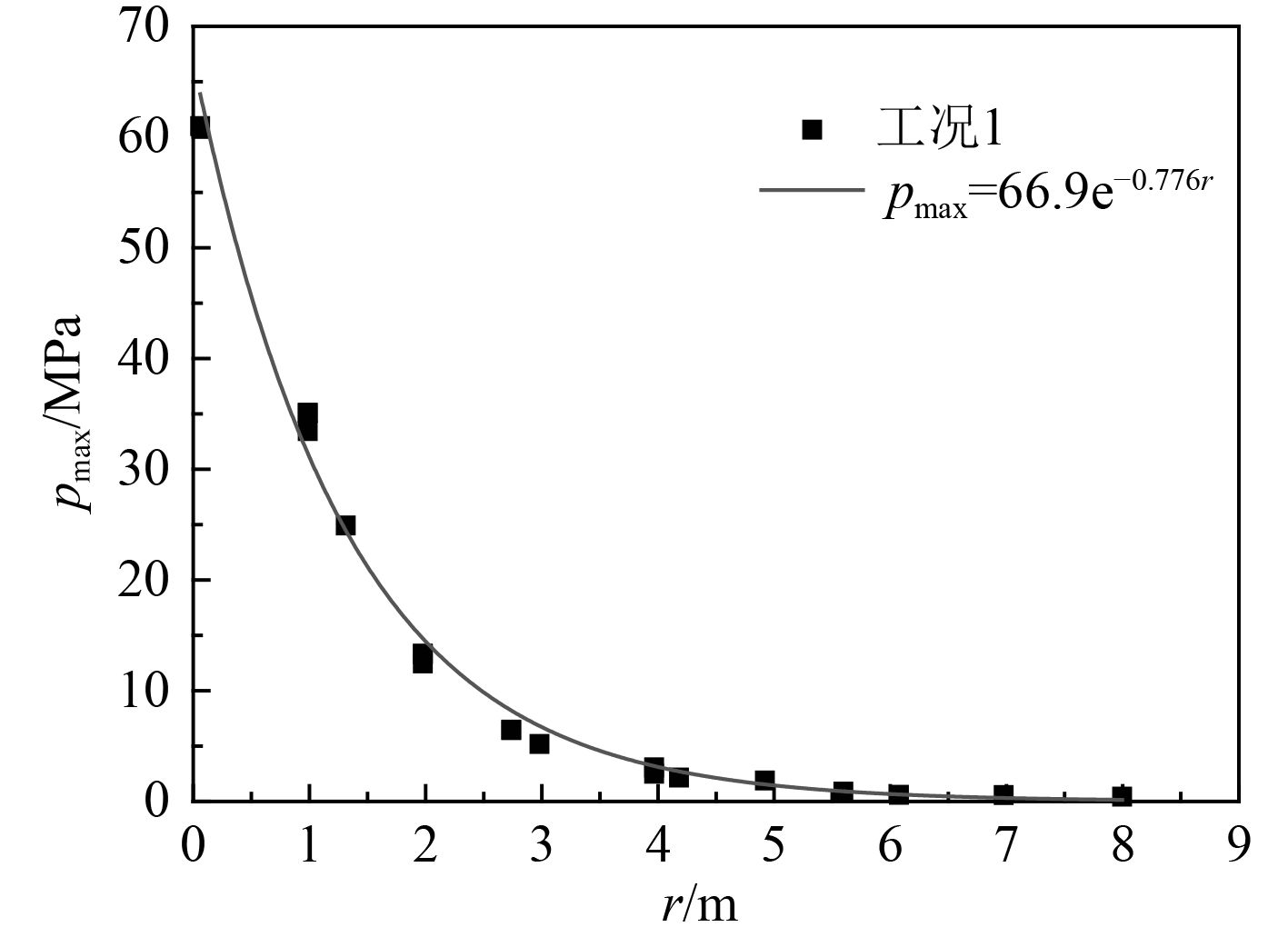图 4 工况1下各测点的压力峰值 Fig. 4 The peak pressure of different points in case 1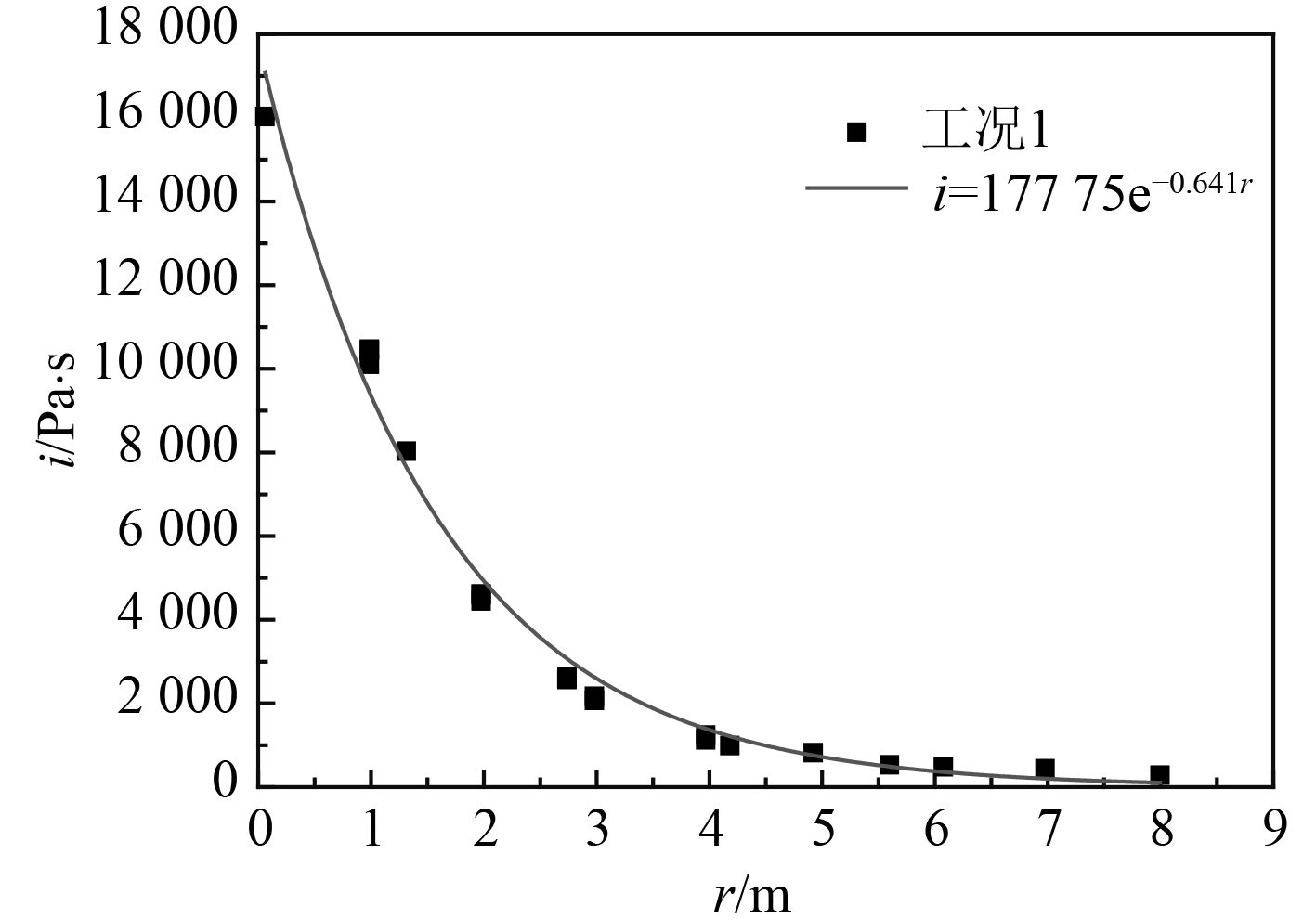图 5 工况1下各测点的比冲量 Fig. 5 The specific impulse of different points in case 1
2.2 破片速度和厚度对防护液舱后板载荷的影响表 2 各工况下趋势线pmax=K1·eαrK1α Tab.2 The K1 and α values of trend line pmax=K1·eαr in different cases表 3 各工况下趋势线i=K2·eβrK2β Tab.3 The K2 and β values of trend line i=K2·eβr in different cases图 6 K1与v的关系 Fig. 6 The relation of K1 and v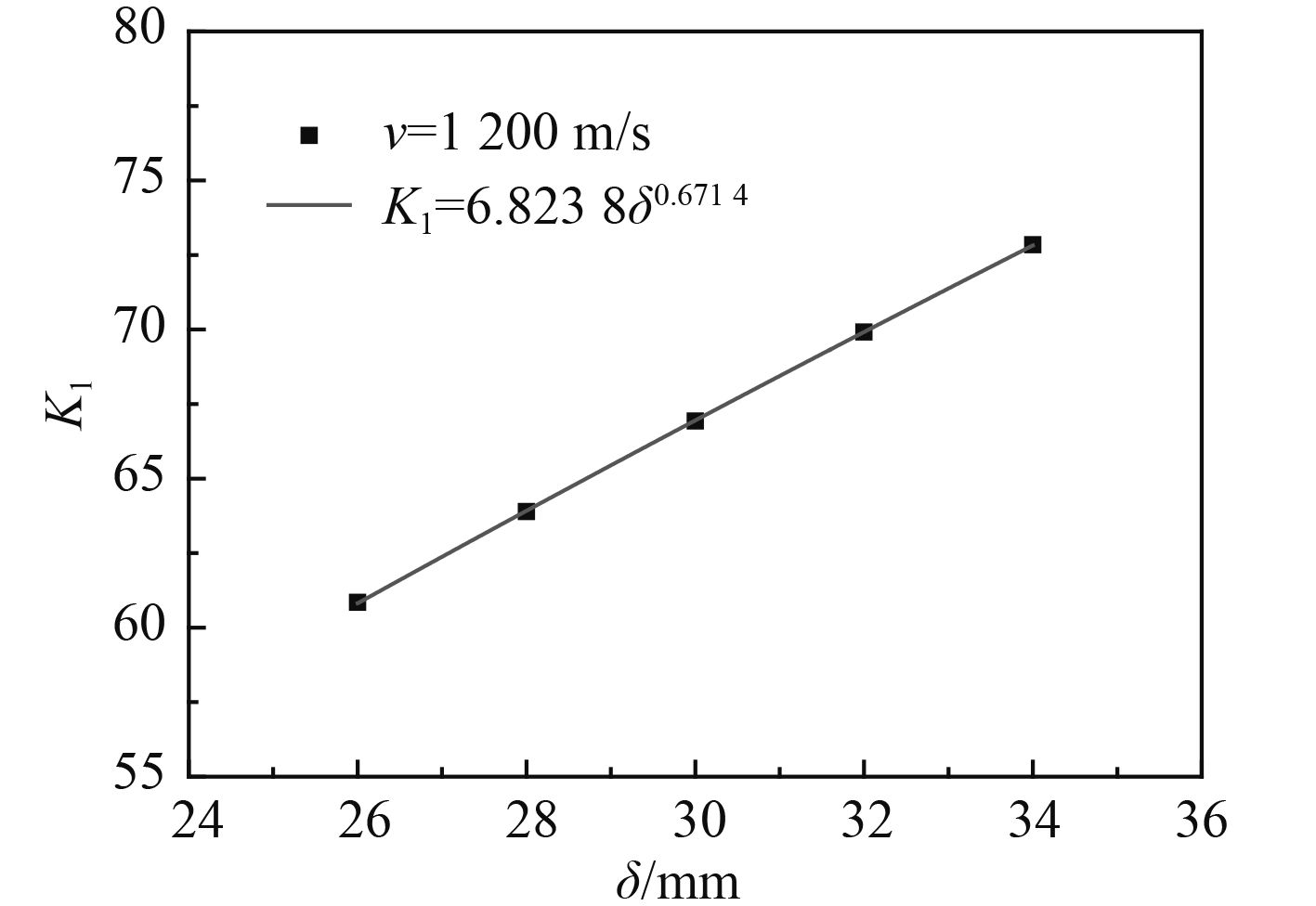图 7 K1与δ的关系 Fig. 7 The relation of K1 and δ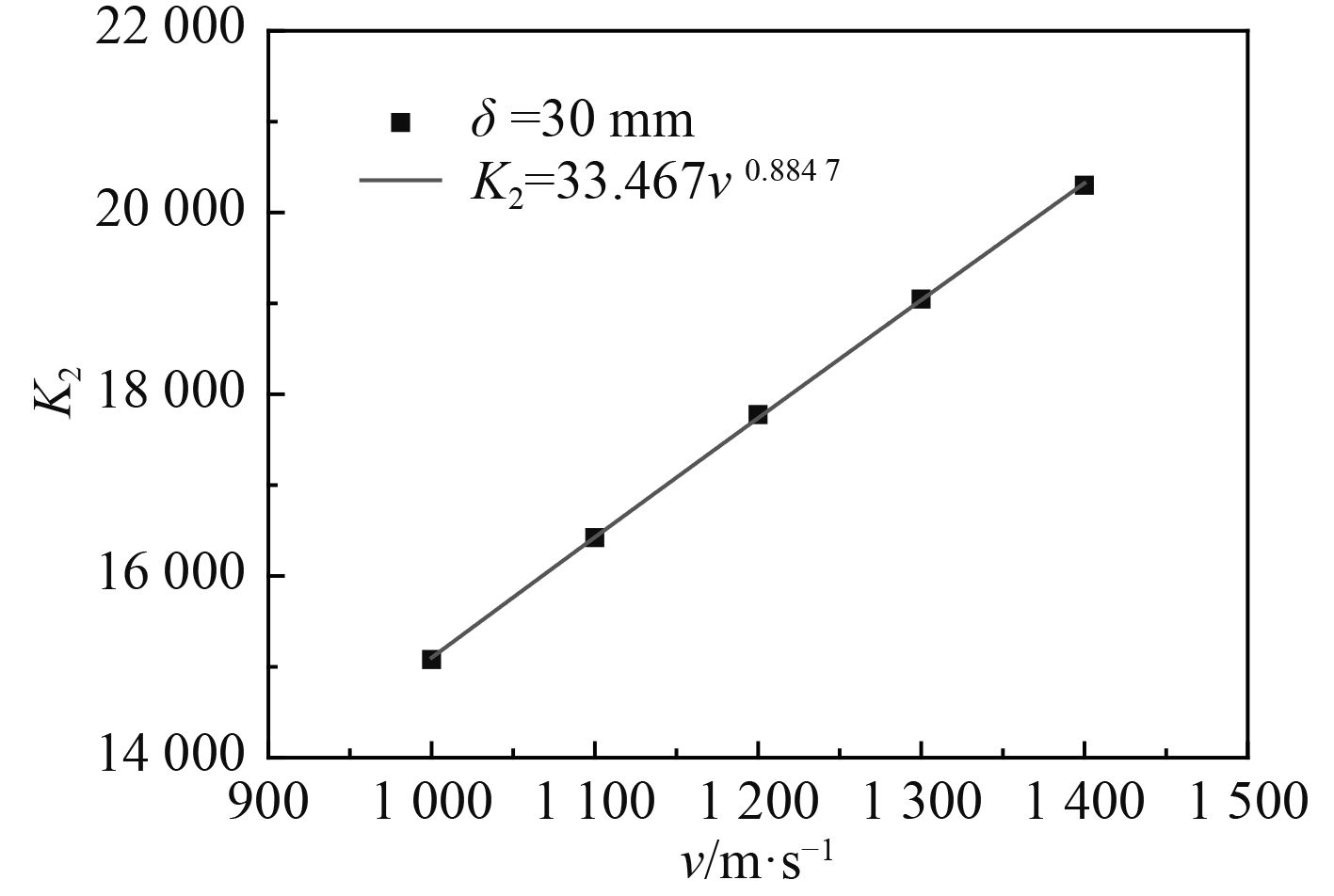图 8 K2与v的关系 Fig. 8 The relation of K2 and v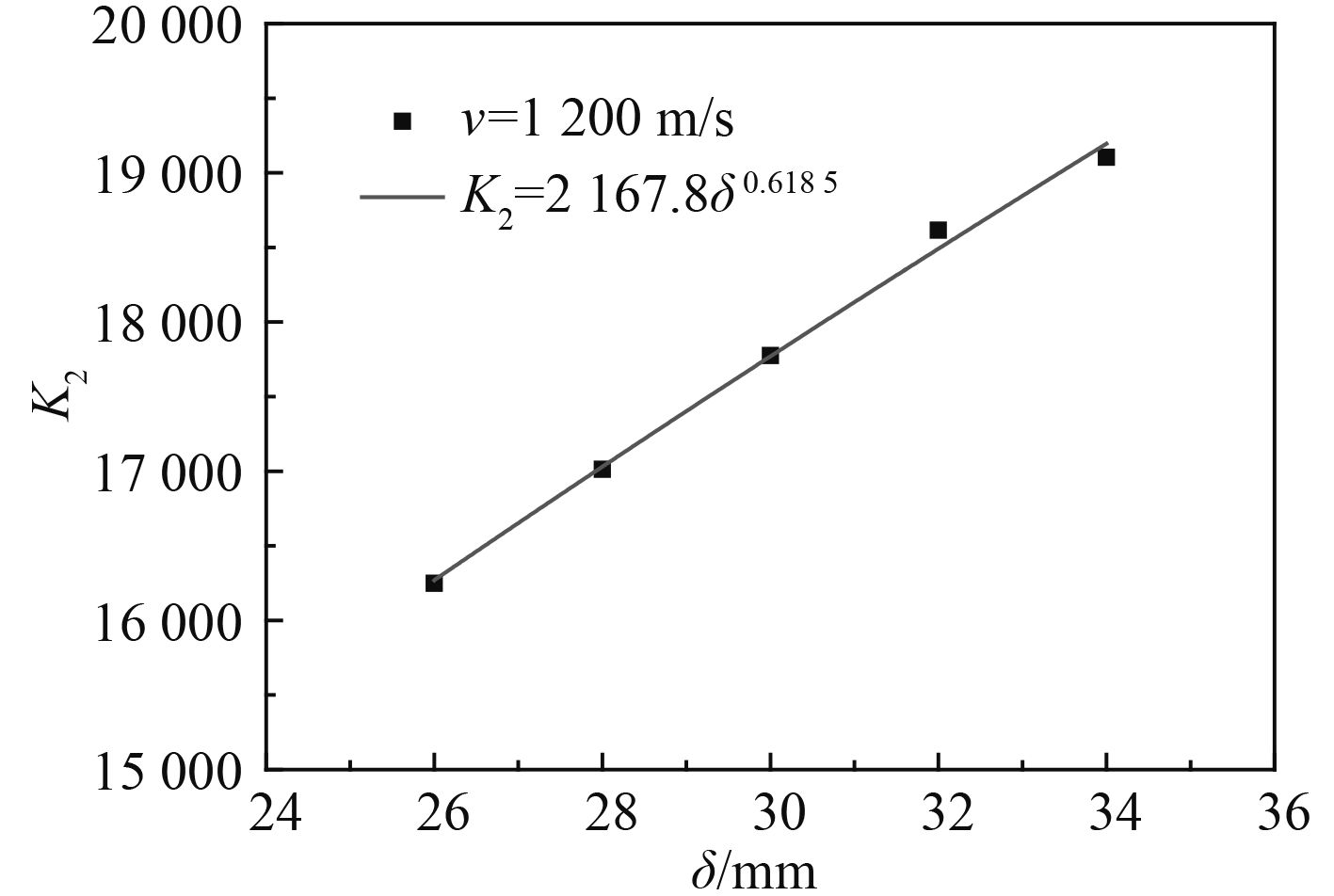图 9 K2与δ的关系 Fig. 9 The relation of K2 and δ
2.3 防护液舱后板载荷拟合公式及验证

 ${p_{\max }} = 0.006 \cdot {v^{0.9927}} \cdot {\delta ^{0.6714}} \cdot {e^{ - 0.767r}}\text{，}$ (4)
 $i = 4.088 \cdot {v^{0.8847}} \cdot {\delta ^{0.6185}} \cdot {e^{ - 0.641r}}\text{。}$ (5)

v=1 050 m/s，δ=27 mm时，液舱后板上各测点的压力峰值pmax和比冲量i的有限元计算值和式（4）、式（5）计算值分别如图10图11所示，两者吻合较好，从而对式（4）、式（5）进行验证。由式（4）、式（5）可看出，破片的速度v比厚度δ对液舱后板载荷的影响更显著。由于式（4）、式（5）是基于有限元计算结果得出的，并且未考虑液舱的宽度、液舱前后板的厚度及装载水的水位等因素对液舱后板载荷的影响，因此在工程应用上式（4）、式（5）有一定的局限性。尽管如此，对文中的防护液舱结构，当破片的速度v和厚度δ在一定范围内变化时，可以利用式（4）、式（5）对其液舱后板的载荷进行计算。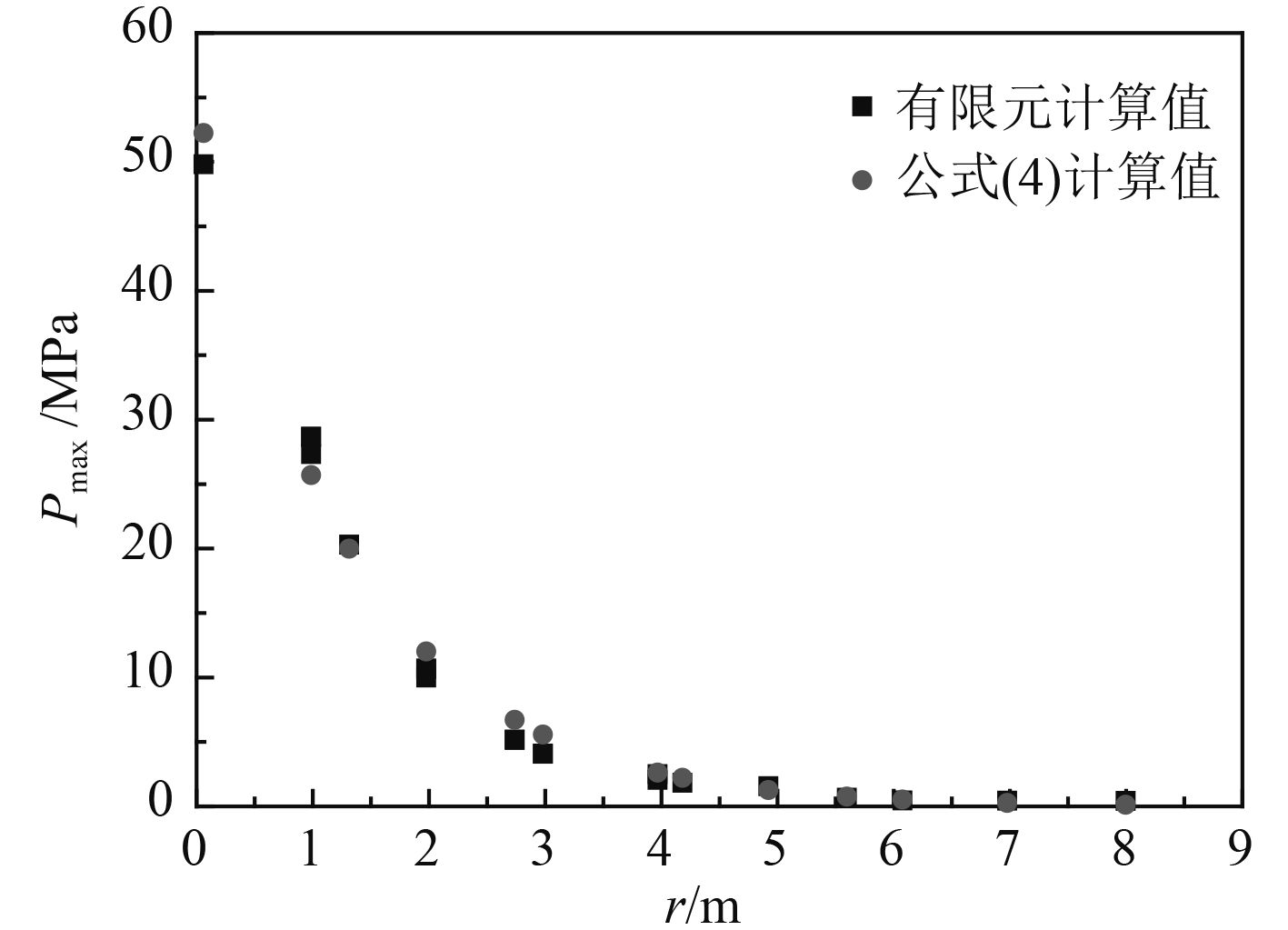图 10 压力峰值（v=1 050 m/s, δ=27 mm） Fig. 10 The peak pressure （v=1 050 m/s, δ=27 mm）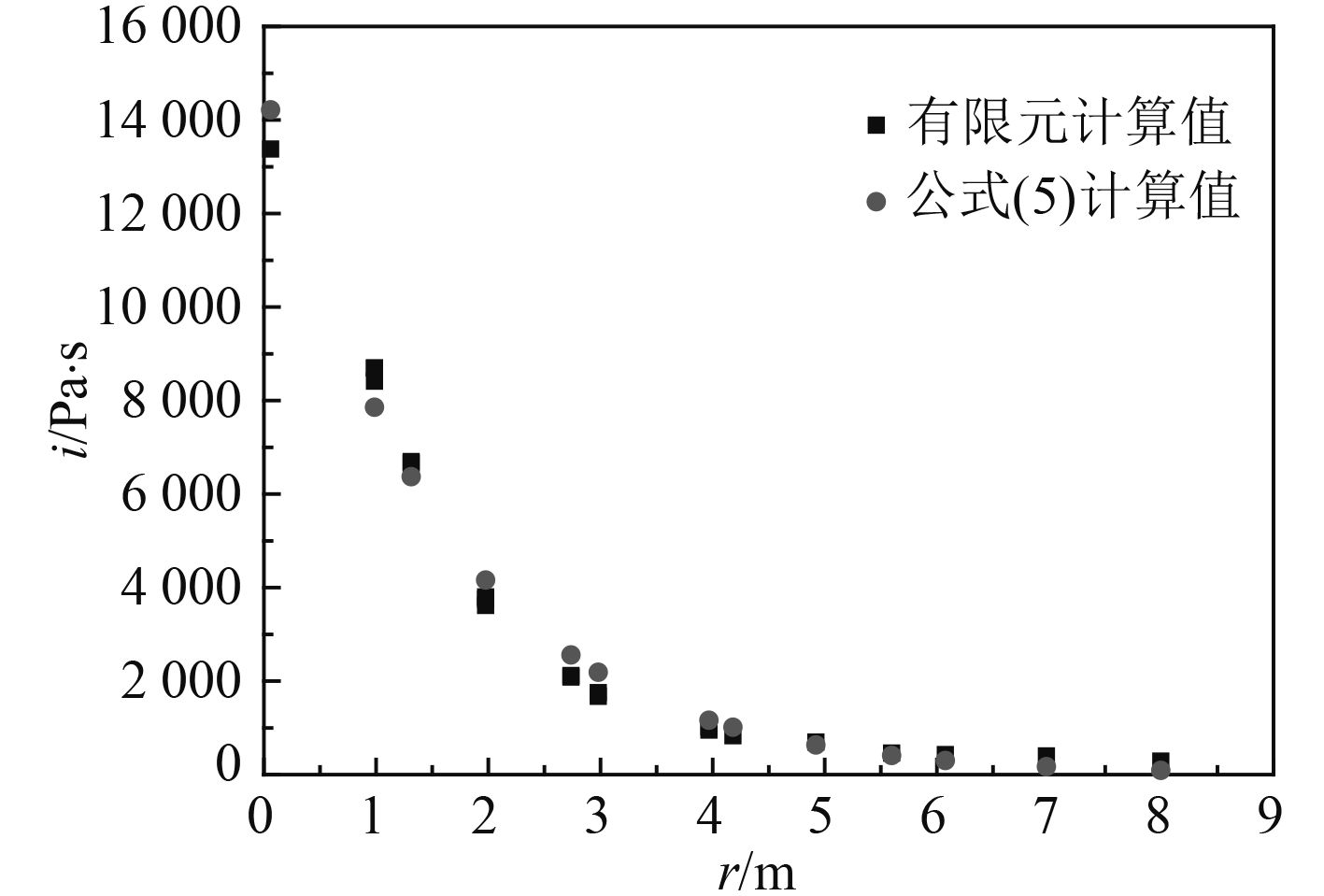图 11 比冲量（v=1 050 m/s, δ=27 mm） Fig. 11 The specific impulse （v=1 050 m/s, δ=27 mm）
3 结　语

1）在空间分布上，液舱后板的载荷在板中心（即破片中心在液舱后板上的投影点）最大，随着到中心点的距离增加而呈指数衰减。

2）增加破片的速度或厚度（或者说增加破片的初始动量），将使液舱后板上任一点的压力峰值和比冲量均增大。

3）文中得到的液舱后板上任一点的压力峰值和比冲量的拟合计算公式可以为防护液舱结构的设计提供参考。

  唐廷, 朱锡, 侯海量, 等. 大型水面舰艇防雷舱结构防护机理数值仿真[J]. 哈尔滨工程大学学报, 2012, 33(2): 142–149. TANG Ting, ZHU Xi, HOU Hai-liang, et al. Numerical simulation study on the defense mechanism of a cabin near the shipboard for large surface vessels[J]. Journal of Harbin Engineering University, 2012, 33(2): 142–149. http://doi.wanfangdata.com.cn/10.3969/j.issn.1006-7043.201012064  VARAS D, LÓPEZ-PUENTE J, ZAERA R. Experimental analysis of fluid-filled aluminium tubes subjected to high-velocity impact[J]. International Journal of Impact Engineering, 2009, 36: 81–91.  VARAS D, ZAERA R, LÓPEZ-PUENTE J. Numerical modelling of the hydrodynamic ram phenomenon[J]. International Journal of Impact Engineering, 2009, 36: 363–374.  PETER J DISIMILE, LUKE A SWANSON, NORMAN TOY. The hydrodynamic ram pressure generated by spherical projectiles[J]. International Journal of Impact Engineering, 2009, 36: 821–829.  VARAS D, ZAERA R, LÓPEZ-PUENTE J. Numerical modelling of partially filled aircraft fuel tanks submitted to hydrodynamic ram[J]. Aerospace Science and Technology, 2012, 16: 19–28.  ARTERO-GUERRERO J A, PERNAS-SÁNCHEZ J, VARAS D, et al. Numerical analysis of CFRP fluid-filled tubes subjected to high-velocity impact[J]. Composite Structures, 2013, 96: 286–297.  徐双喜, 吴卫国, 李晓彬, 等. 舰船舷侧防护液舱舱壁对爆炸破片的防御作用[J]. 爆炸与冲击, 2010, 30(4): 395–400. XU Shuang-xi, WU Wei-guo, LI Xiao-bin, et al. Protective effect of guarding fluid cabin bulkhead under attacking by explosion fragments[J]. Explosion and Shock Waves, 2010, 30(4): 395–400.  沈晓乐, 朱锡, 侯海量, 等. 高速破片侵彻防护液舱试验研究[J]. 中国舰船研究, 2011, 6(3): 12–15. SHEN Xiao-le, ZHU Xi, HOU Hai-liang, et al. Experimental study on penetration properties of high velocity fragment into safety liquid cabin[J]. Chinese Journal of Ship Research, 2011, 6(3): 12–15. http://www.cqvip.com/QK/93256A/201103/38474331.html  孔祥韶, 吴卫国, 李俊, 等. 爆炸破片对防护液舱的穿透效应[J]. 爆炸与冲击, 2013, 33(5): 471–478. KONG Xiang-shao, WU Wei-guo, LI Jun, et al. Effects of explosion fragments penetrating defensive liquid-filled cabins[J]. Explosion and Shock Waves, 2013, 33(5): 471–478.  孔祥韶, 吴卫国, 刘芳, 等. 舰船舷侧防护液舱对爆炸破片的防御作用研究[J]. 船舶力学, 2014, 18(8): 996–1004. KONG Xiang-shao, WU Wei-guo, LIU Fang, et al. Research on protective effect of guarding fluid cabin under attacking by explosion fragments[J]. Journal of Ship Mechanics, 2014, 18(8): 996–1004. http://www.wanfangdata.com.cn/details/detail.do?_type=perio&id=cblx201408015  张元豪, 陈长海, 侯海量, 等. 高速破片侵彻防护液舱后的水中运动特性试验研究[J]. 兵器材料科学与工程, 2016, 39(5): 44–48. ZHANG Yuan-hao, CHEN Chang-hai, HOU Hai-liang, et al. Experimental study on kinetic characteristic of high velocity fragments in water after penetration of protecting liquid cabin[J]. Ordnance Material Science and Engineering, 2016, 39(5): 44–48. http://www.cnki.com.cn/Article/CJFDTOTAL-BCKG201605013.htm  张婧. 舰船结构在爆炸作用下的非线性响应及可靠性研究[D]. 哈尔滨: 哈尔滨工程大学, 2009.  吴林杰, 朱锡, 侯海量, 等. 空中近距爆炸下加筋板架的毁伤模式仿真研究[J]. 振动与冲击, 2013, 32(14): 77–81. WU Lin-jie, ZHU Xi, HOU Hai-liang, et al. Simulations for damage modes of a stiffened plate subjected to close-range air-blast loading[J]. Journal of Vibration and Shock, 2013, 32(14): 77–81.